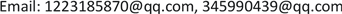SAStatistics and Application2325-2251Scientific Research Publishing10.12677/SA.2019.82038SA-29810SA20190200000_72289931.pdf数学与物理 关于海南省商品住宅价格模型的分析与预测 Analysis and Forecast of Commodity Housing Price Model in Hainan Province 21疏桐21山东科技大学，山东 青岛null290320190802341357© Copyright 2014 by authors and Scientific Research Publishing Inc. 2014This work is licensed under the Creative Commons Attribution International License (CC BY). http://creativecommons.org/licenses/by/4.0/Copyright © 2019 by authors and Hans Publishers Inc.

This work is licensed under the Creative Commons Attribution International License (CC BY).

http://creativecommons.org/licenses/by/4.0/1. 引言

1) 搜集相关数据，找出决定海南省主要城市商品住宅价格的影响因素，进行相关定性定量分析，并分析各种因素之间的关系。

2) 假设政府没有出台限购政策，结合问题二中建立的数学模型，按照月份预测2018年6月~2019年5月海南省主要城市商品住宅价格。

3) 若考虑2018年4月22日限购政策出台，重新建立数学模型，按照月份预测2018年6月~2019年5月海南省主要城市商品住宅价格。

2. 问题说明

(I) 符号假定

1) 海口城市：

x 1 ：居民人均可支配收入；

x 2 ：城市经济发展；

x 3 ：旅游业；

y ：商品住宅价格。

2) 三亚城市：

：居民人均可支配收入；

x 2 ：城市经济发展；

x 3 ：城市经济发展；

x 4 ：房屋造价；

y ：商品住宅价格。

(II) 模型假设

1) 假定搜集到的数据具有一定的可靠性和真实性；

2) 数据预测时期假定没有出台各种相关政策，受到其他因素的影响都在可控范围内；

3) 海口和三亚两个城市对于海南省有较好的代表性。

(III) 数据预处理

1) 在相关网站上搜集爬取有关数据，并进行统计整理；

2) 使用MATLAB软件，对各种指标的单位进行标准化处理，以消除量纲对模型的影响。

3. 模型的建立与求解3.1. 问题一分析海南省商品住宅价格的影响因素3.1.1. 海南省主要城市商品住宅价格变化总体趋势

3.1.2. 商品住宅价格影响因素的定性分析

1) 国家宏观政策：

2009年，国务院正式批复《国务院关于推进海南国际旅游岛建设发展的若干意见》，2010年，史上最严的房地产调控政策“新国十条”发布，但海南省受国际旅游岛概念影响，旅游业大幅度发展，游客对房地产需求增大，导致房价大幅上涨。2011年，楼市“三限”、一房一价出台，国家调控力度加大，全国房价继续上涨，海南省房价回落，降为近十年最低价格。2012、2013年，海南省要求清理楼市库存，商品住宅价格下行明显，开发商开始以价换量。

2014年，三亚停建80平以下户型，海口限购松绑，新政出台后，全国楼市普遍迎来成交高峰，但海南成交持续下行。2015、2016年，海南省停建60平以下小户型，中国人民银行降准降息，海南下调住房公积金存贷款利率，海南实施“两个暂停”政策，购房者回归理性，一线城市爆发涨价潮，海南依然以去库存为主，全省库存大幅下降，量价普涨  。

2) 交通发展：

3) 消费者理性预期心理因素：

3.1.3. 商品住宅价格影响因素的定量分析

(I) 海口城市各相关因素变化情况

(II) 三亚城市各相关因素变化情况

3.2. 构建商品住宅价格数学模型3.2.1. 初步分析商品住宅价格的线性相关模型

1) 模型建立

y = β 0 + β 1 x 1 + β 2 x 2 + β 3 x 3

2) 模型求解

Test statisti

15,208[−14,000, 44,417]
β 1−1[−9, 6]
β 213[561, 587]
β 3162[162, 414]
R 2 = 0.3313， F = 111， P < 0.1259， S 2 = 2.0372

3) 残差分析

3.2.2. 自变量多重共线性的处理

(I) 海口市共线性处理

1) 相关性检验

Test of KMO and Bartlet

Bartlett的球形度检验近似卡方43.711
df3
Sig.0.000

a) 提取主成分和公因子

Total variance explaine

12.96598.83698.8362.96598.83698.836
20.0331.09199.927
30.0020.073100.000

2) 计算正交化单位特征向量

l i = λ i e i ( i = 1 , 2 , 3 )

3) 建立回归方程并拟合其相关性

Component matri

1

a. 已提取了1个成份。

Principal component eigenvector valu
e 10.580
e 20.578
e 30.43

Coefficien

B标准误Beta

(常数)1.000E−0130.2460.0001.000

Model summar
RR方调整R方估计值的标准误
0.9560.9140.518778.514

y = 0 .766 X

y = 0.444 x 1 + 0.443 x 2 + 0.329 x 3

(II) 三亚

Unit eigenvecto
e 10.52
e 20.52
e 30.52
e 40.43

Coefficien

B标准误Beta

(常数)−1.000E−0130.1490.0001.000

Model summar
RR方调整R方估计值的标准误
0.9330.8700.8441512.704

y = 0 .933 X

y = 0.48516 ( x 1 + x 2 + x 3 ) + 0.40119 x 4

3.2.3. 模型的最终确定

3.3. 按月分析2018年6月~2019年5月海南省商品住宅房价格3.3.1. 粗糙集的划分

Indicators of Hainan province in the past year

2009000010
2010000010
2011010110
2012111010
2013111111
2014110111
2015111111
2016111111

Pos _ c { D } = { 2013 , 2014 , 2015 , 2016 }

Pos _ ( c − c 2 ) { D } = { 2011 , 2013 , 2014 } = Pos _ c { D }

Pos _ ( c − c 3 ) { D } = { 2012 , 2015 , 2016 } = Pos _ c { D }

3.3.2. 灰色模型GM(1, 1)建立

d X ( 1 ) d t + a X ( 1 ) = μ (5.12)

δ = ( B T B ) ( − 1 ) B T Y n (5.13)

X ( 1 ) ( k ^ + 1 ) = [ X ( 0 ) ( 1 ) − μ a ] ⋅ e − a k + μ a ( k = 0 , 1 , 2 ⋯ , n ) (5.14)

3.3.3. 模型检验校正

ρ ( k ) = 1 − ( 1 − 0.5 a 1 + 0.5 a ) λ (k)

3.3.4. 模型求解

1) 数据检验和预处理

λ ( k ) = x ( 0 ) ( k − 1 ) x ( 0 ) ( k ) , k = 2 , 3 , ⋯ , n .

2) 利用matlab编程进行GM(1, 1)建模

δ 1 = [ − 0.034 13.185 ] , y 1 = 405.085 * e 0.0342791 * t − 384.645 δ 2 = [ − 0.008 2442.56 ] , y 2 = 325800 * e 0.0075673 * t − 322772 δ 3 = [ 0.0095 113.909 ] , y 3 = − 11919.2 * e − 0.00945915 * t + 12042.2

Prediction of data based on gray-scale mode

GDP125.97122.18117.36117.48117.91118.03

GDP123.14139.66135.39134.70128.14131.68

3) 模型的检验

3.3.5. 模型的代入

1) 海口

Forecast of house price in Haikou cit

2) 三亚

Forecast of house price in Sanya cit

3.4. 按月分析政策出台后2018年6月~2019年5月海南省商品住宅房价格3.4.1. 数据预处理及海口市VAR模型的构建

VAR模型对于相互联系的时间序列变量系统是有效的预测模型，对于政策虚拟变量，本问题将运用VAR模型来研究其对商品住宅价格的动态影响。参考文献  ，为减小数据序列的异方差影响，首先对政策虚拟变量取自然对数，得到ln(P)的一个新的时间序列数据。从而建立滞后K期的VAR模型如下：

( ln Price t ln P t ln T t lnGDP t ) = c + ∑ i = 1 k ( ln Price t − 1 ln P t − 2 ln T t − 3 lnGDP t 3 ) + ( u 1 u 2 u 3 u 4 )

3.4.2. 脉冲函数响应分析

Impluses只留P (政策虚拟变量)，得到脉冲曲线图13。

3.4.3. 商品住宅价格预测

Δ ln Price t = ( 1.231831 82.31819 − 0.365957 0.247278 ) T ( ln Price t − 1 Δ ln P t − 1 Δ ln T t − 1 Δ ln GDP t − 1 ) + ( − 0.194865 − 148.1100 10.97197 1.030293 ) T ( Δ ln Price t − 2 Δ ln P t − 2 Δ ln T t − 2 Δ ln GDP t − 2 ) − 384.6593

Δ ln Price t = ( 0.917230 − 649.9250 3.401510 2.948141 ) T ( ln Price t − 1 Δ ln P t − 1 Δ ln T t − 1 Δ ln GDP t − 1 ) + ( 0.184801 463.2524 13.73099 0.261242 ) T ( Δ ln Price t − 2 Δ ln P t − 2 Δ ln T t − 2 Δ ln GDP t − 2 ) − 2143.552

3.4.4. 模型预测

3.4.5. 结合数据定性分析政策影响